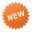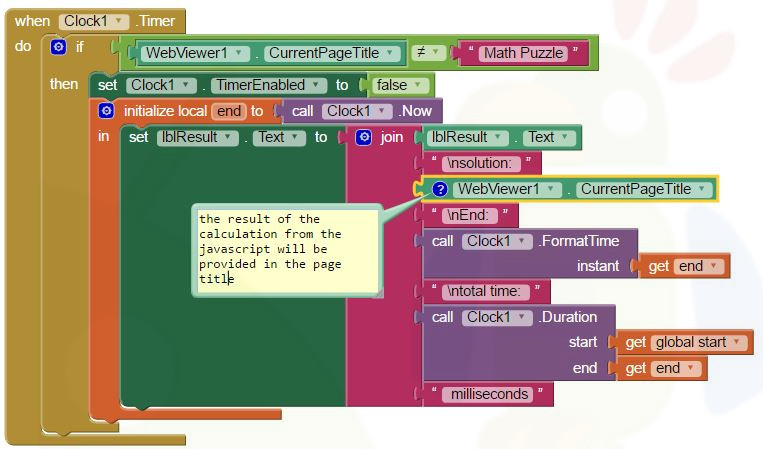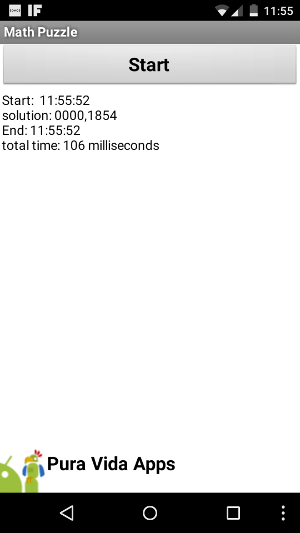#App Inventor and embedded HTML/JavaScript

## App Inventor is noin calculations anymore!

Let's do a math puzzle, for example this one from Fun With Num3ers. I will repeat the puzzle here:

```        Find a 4-digit Num3er   abcd   such that

(1)   a + b   =   c + d
the sum of the first two digits is equal to that of the last two digits.

(2)   a + d   =   c
The sum of the first and last digits is equal to the third digit.

(3)   b + d   =   2 * (a + c)
the sum of the second and fourth digits is twice the sum of the other two digits.
```

Try to solve that with App Inventor only! How many minutes do you have to wait to get a result? Now take a look at the embedded HTML/Javascript solution and you will see, that it takes less than 1 second to do the calculation!

The HTML file math.html is uploaded as asset to App Inventor.

There is one special thing to consider for HTML documents uploaded as assets into App Inventor: During development, you have to use the development path to the embedded HTML document.

`file:///mnt/sdcard/AppInventor/assets/math.html`

Before packaging the app, use the production path.

`file:///android_asset/math.html`The tools extension offers the method PathToAssets which always returns the correct path to the assets.

### App Inventor Blocks### Screenshot### HTML/JavaScript

```<!DOCTYPE html>
<html>
<meta name="author" content="puravidaapps.com">
<meta charset="utf-8">
<meta name="viewport" content="width=device-width, initial-scale=1">
<title>Math Puzzle</title>

<body>
```
```  <script>
var intCounter = 0;
var strResult  = '';

while (intCounter <= 9999) {
intDigit = 10000 + intCounter;

// this is a 4 digit string with leading zeros
strDigit = intDigit.toString().substr(1, 4);

// these are the individual digits
a = parseInt(strDigit.substr(0, 1));
b = parseInt(strDigit.substr(1, 1));
c = parseInt(strDigit.substr(2, 1));
d = parseInt(strDigit.substr(3, 1));

// these are the rules
if ((a + b == c + d) && (a + d == c) && (b + d == 2 * (a + c))) {
strResult = strResult+strDigit + ',';
}

intCounter++;
}
// print the result to the page title, remove the last comma
window.document.title= strResult.substr(0, strResult.length-1)
</script>
```
```</body>
</html>
```

Developing and maintaining snippets, tutorials and extensions for App Inventor takes a lot of time.
I hope it saved some of your time. If yes, then you might consider to donate a small amount!

Donation amount:or donate some mBTC toAddress:
1Jd8kXLHu2Vkuhi15TWHiQm4uE9AGPYxi8Thank you! Taifun